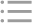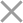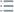## Fill out the form in 2 simple steps below:

Step 1

Step 2
** By clicking this button you agree to our Privacy Policy statementRequest our prices
--Select currency--
USD
EUR
* By clicking this button you agree to our Privacy Policy statementFree PVS‑Studio license for Microsoft MVP specialists
* By clicking this button you agree to our Privacy Policy statementTo get the licence for your open-source project, please fill out this form
* By clicking this button you agree to our Privacy Policy statementI am interested to try it on the platforms:
 Windows Linux macOS PVS-Studio for .NET Core JetBrains Rider
* By clicking this button you agree to our Privacy Policy statementMessage submitted.

Your message has been sent. We will email you at

check your Spam/Junk folder and click the "Not Spam" button for our message.
This way, you won't miss messages from our team in the future.

>
>
>
V826. Consider replacing standard conta…Introduction
Analyzing projects
On Windows
On Linux and macOS
Cross-platform
IDE
Build systems
Game Engines
Continuous use of the analyzer in software development
Deploying the analyzer in cloud Continuous Integration services
Managing analysis results
Analyzer diagnostics
General Analysis (C++)
General Analysis (C#)
General Analysis (Java)
Micro-Optimizations (C++)
Micro-Optimizations (C#)
Diagnosis of 64-bit errors (Viva64, C++)
Customer specific requests (C++)
MISRA errors
AUTOSAR errors
OWASP errors (C++)
OWASP errors (C#)
OWASP errors (Java)
Problems related to code analyzerContents

# V826. Consider replacing standard container with a different one.

Jul 21 2020

The analyzer has detected a standard C++ library container that can be replaced with another one for optimization.

To determine which container type will suit better in a given case, heuristics are used based on what operations are performed on the container. The analyzer also calculates algorithmic complexity of all the operations and suggests a container whose algorithmic complexity is lowest.

The warning message will briefly describe the reason for suggesting the replacement:

• "The size is known at compile time" – the container's size is known at compile time, so it can be replaced with a static array (std::array).
• "Elements are added, read and erased only from front/back" – the container implements a LIFO queue, so it can be replaced with 'std::stack'.
• "Elements are added to front/back, read and erased from the opposite side" – the container implements a FIFO queue, so it can be replaced with 'std::queue'.
• "Insertion and removal of elements occur at either side of the container" – elements are added or removed at either head or tail of the container. In this case, 'std::deque' or 'std::list' will be an efficient substitute.
• "Insertions occur at the front side, and the container is traversed forward" – elements are added only to the beginning of the container and the container is traversed forward. In this case, it is used as 'std::forward_list'.
• "Insertions occur at the back side, and the container is traversed" – elements are added only to the end of the container and the container is traversed in any direction. In this case, 'std::vector' will be the most efficient substitute.
• "Contiguous placement of elements in memory can be more efficient" – using 'std::vector' may enhance performance due to contiguous placement of elements in memory without increasing algorithmic complexity.
• "Increased overall efficiency of operations" – the container type was chosen based on statistical analysis.

Consider the following example:

``````void f()
{
std::vector<int> v;
v.push_back(1);
v.push_back(2);
v.push_back(3);

for (auto value : v)
{
std::cout << value << ' ';
}
}``````

The analyzer issues the following message:

V826. Consider replacing the 'v' std::vector with std::array. The size is known at compile time.

The vector's size is known at compile time. We can use 'std::array' instead to avoid dynamic allocation. Optimized version:

``````void f()
{
std::array a{1, 2, 3};
}``````

The analyzer will not suggest the replacement if the total size of the vector's elements exceeds 16 Kbytes or if the vector is passed to the function or returned from it or passed as an argument to another function.

In the following example, the analyzer will keep silent even though the container's size is known at compile time:

``````std::vector<int> f()
{
std::vector<int> v;
v.push_back(1);
v.push_back(2);
v.push_back(3);

return v;
}``````

Another example of code that can be optimized:

``````void f(int n)
{
std::vector<int> v;

for (int i = 0; i < n; ++i)
{
v.push_back(i);
}

for (int i = 0; i < n; ++i)
{
std::cout << v.back() << ' ';
v.pop_back();
}
}``````

The analyzer issues the following message:

V826. Consider replacing the 'v' std::vector with std::stack. Elements are added, read and erased only from front/back.

In this case, elements are added at the end of the vector, then read sequentially and removed. The vector is used as 'std::stack', so it can be replaced with this type of container. Optimized version:

``````void f(int n)
{
std::stack<int> v;

for (int i = 0; i < n; ++i)
{
v.push(i);
}

for (int i = 0; i < n; ++i)
{
std::cout << v.top() << ' ';
v.pop();
}
}``````

Another example of code that can be optimized:

``````void f(int n)
{
std::deque<int> d;
for (int i = 0; i < n; i++)
{
d.push_back(i);
}

for (auto value : d)
{
std::cout << value << ' ';
}
}``````

The analyzer issues the following message:

V826. Consider replacing the 'd' std::deque with std::vector. Contiguous placement of elements in memory can be more efficient.

In this case, 'std::deque' and 'std::vector' are equivalent substitutes in terms of algorithmic complexity. However, in a vector, the elements will be placed sequentially, which may help increase performance since sequential memory access enables more efficient use of the CPU cache. Optimized version:

``````void f(int n)
{
std::vector<int> d;
for (int i = 0; i < n; i++)
{
d.push_back(i);
}

for (auto value : d)
{
std::cout << value << ' ';
}
}``````